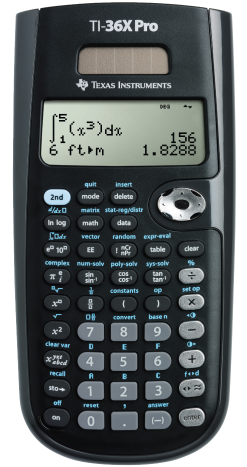Navigate: Home / Hardware / Calculators / Scientific Calculators / Texas Instruments TI-36X Pro Scientific Calculator

Texas Instruments TI-36X Pro Scientific Calculator

Ideal for computer science and engineering courses in which graphing technology may not be permitted.
*Price: \$24.95
 SKU: TI36XPRO-X Mfr Part #: Other: 36PRO/TBL/1L1/A Requires Academic ID: No
Limit one sharing discount per purchase.
 The TI-36X Pro Scientific Calculator is an advanced, four-line scientific calculator, with higher-level math and science functionality, is ideal for computer science and engineering courses in which graphing technology may not be permitted.The TI-36X Pro scientific calculator is approved for use on SAT®, ACT®, and AP® exams.Features include: View Multiple Calculations at One TimeCompare results and explore patterns on-screen with the MultiView™ display, which supports up to four lines. See Math Exactly as it Appears in TextbooksSee math expressions, symbols and stacked fractions exactly the way they appear in textbooks — no need to adapt to a technical syntax; also provides quick access to frequently used functions. Built-In SolversIntuitive prompts allow one to easily solve numeric equation, polynomial and system of linear equations. Easy-to-Use Mode MenuThe menu is structured for easy, intuitive access to commands; select degrees/radians, floating/fix, Classic/MathPrint™ feature or number format. Explore (x,y) Tables of ValuesStudents can easily explore an (x,y) table of values for a given function, automatically or by entering specific x values.Key Features:Four-line displayOne- and two-variable statisticsMultiView™ display shows multiple calculations at the same time on screenSelect degrees/radians, floating/fix, number format modesChoose from three solvers: numeric equation, polynomial and system of linear equationsDisplay a defined function in a tabular formDetermine the numeric derivative and integral for real functionsPerform vectors and matrices using a vector and matrix entry windowIdeal For:Algebra I and IIGeometryTrigonometryStatisticsCalculusBiologyChemistryPhysicsComputer scienceCollege mathCollege scienceCollege engineering